# Selina Concise Mathematics Class 8 ICSE Solutions Chapter 14 Linear Equations in one Variable

## Selina Concise Mathematics Class 8 ICSE Solutions Chapter 14 Linear Equations in one Variable (With Problems Based on Linear equations)

Selina Publishers Concise Mathematics Class 8 ICSE Solutions Chapter 14 Linear Equations in one Variable (With Problems Based on Linear equations)

### Linear Equations in one Variable Exercise 14A – Selina Concise Mathematics Class 8 ICSE Solutions

Solve the following equations:
Question 1.
20 = 6 + 2x
Solution: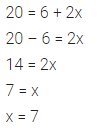Question 2.
15 + x = 5x + 3
Solution:Question 3.
$$\frac { 3x+2 }{ x-6 }$$ = -7
Solution:Question 4.
3a – 4 = 2(4 – a)
Solution: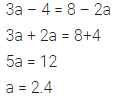Question 5.
3(b – 4) = 2(4 – b)
Solution: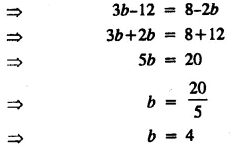Question 6.
$$\frac { x+2 }{ 9 } =\frac { x+4 }{ 11 }$$
Solution:Question 7.
$$\frac { x-8 }{ 5 } =\frac { x-12 }{ 9 }$$
Solution: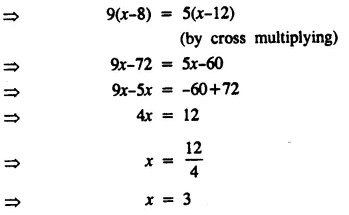Question 8.
5(8x + 3) = 9(4x + 7)
Solution:Question 9.
3(x + 1) = 12 + 4(x – 1)
Solution: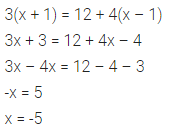Question 10.
$$\frac { 3x }{ 4 } -\frac { 1 }{ 4 } \left( x-20 \right) =\frac { x }{ 4 } +32$$
Solution: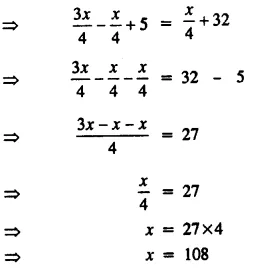Question 11.
$$3a-\frac { 1 }{ 5 } =\frac { a }{ 5 } +5\frac { 2 }{ 5 }$$
Solution:Question 12.
$$\frac { x }{ 3 } -2\frac { 1 }{ 2 } =\frac { 4x }{ 9 } -\frac { 2x }{ 3 }$$
Solution: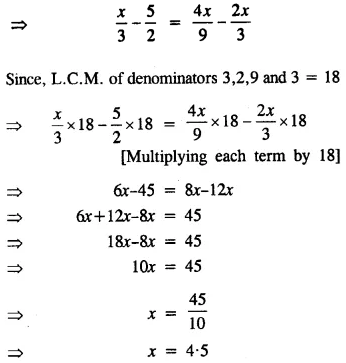Question 13.
$$\frac { 4\left( y+2 \right) }{ 5 } =7+\frac { 5y }{ 13 }$$
Solution: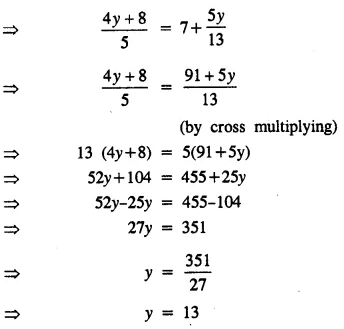Question 14.
$$\frac { a+5 }{ 6 } -\frac { a+1 }{ 9 } =\frac { a+3 }{ 4 }$$
Solution: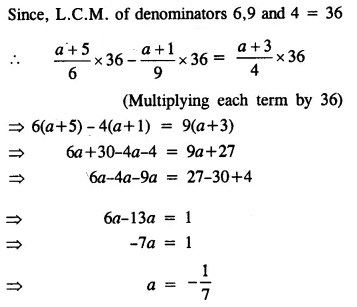Question 15.
$$\frac { 2x-13 }{ 5 } -\frac { x-3 }{ 11 } =\frac { x-9 }{ 5 } +1$$
Solution:Question 16.
6(6x – 5) – 5 (7x – 8) = 12 (4 – x) + 1
Solution: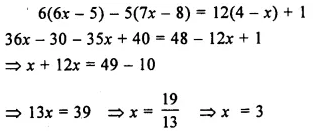Question 17.
(x – 5) (x + 3) = (x – 7) (x + 4)
Solution:Question 18.
(x – 5)2 – (x + 2)2 = -2
Solution:Question 19.
(x – 1) (x + 6) – (x – 2) (x – 3) = 3
Solution: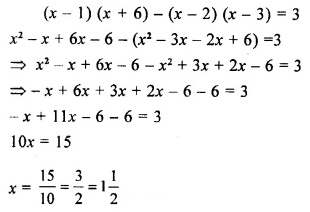Question 20.
$$\frac { 3x }{ x+6 } -\frac { x }{ x+5 } =2$$
Solution:Question 21.
$$\frac { 1 }{ x-1 } +\frac { 2 }{ x-2 } =\frac { 3 }{ x-3 }$$
Solution: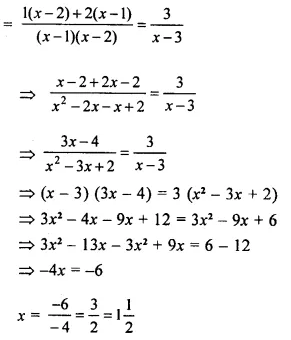Question 22.
$$\frac { x-1 }{ 7x-14 } =\frac { x-3 }{ 7x-26 }$$
Solution: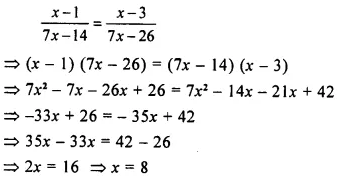Question 23.
$$\frac { 1 }{ x-1 } -\frac { 1 }{ x } =\frac { 1 }{ x+3 } -\frac { 1 }{ x+4 }$$
Solution: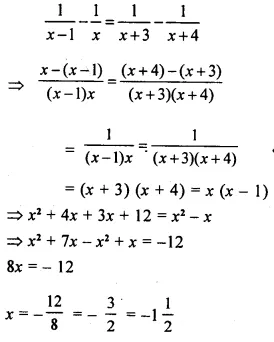Question 24.
Solve: $$\frac { 2x }{ 3 } -\frac { x-1 }{ 6 } +\frac { 7x-1 }{ 4 } =2\frac { 1 }{ 6 }$$
Hence, find the value of ‘a’, if $$\frac { 1 }{ a }$$ + 5x = 8.
Solution: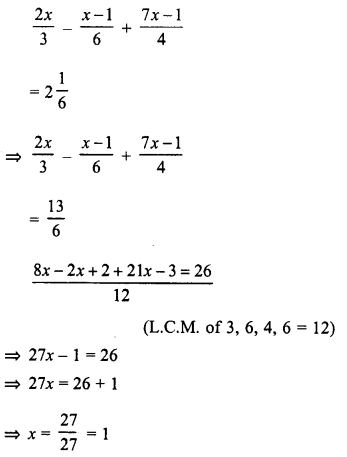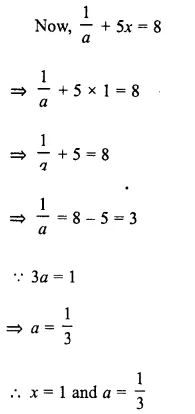Question 25.
Solve: $$\frac { 4-3x }{ 5 } +\frac { 7-x }{ 3 } +4\frac { 1 }{ 3 } =0$$
Hence find the value of ‘p’ if 2p – 2x + 1 = 0
Solution: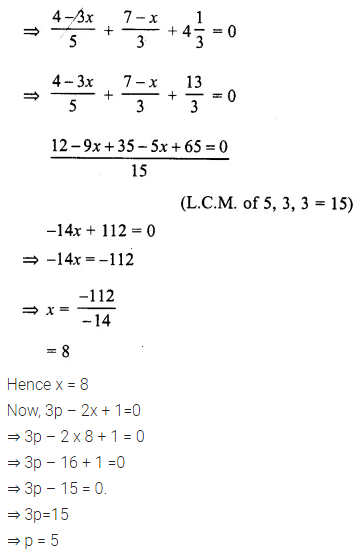Question 26.
Solve: $$0.25+\frac { 1.95 }{ x } =0.9$$
Solution:Question 27.
Solve: $$5x-\left( 4x+\frac { 5x-4 }{ 7 } \right) =\frac { 4x-14 }{ 3 }$$
Solution: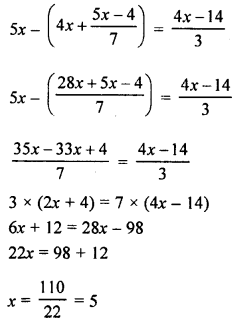### Linear Equations in one Variable Exercise 14B – Selina Concise Mathematics Class 8 ICSE Solutions

Question 1.
Fifteen less than 4 times a number is 9. Find the number.
Solution: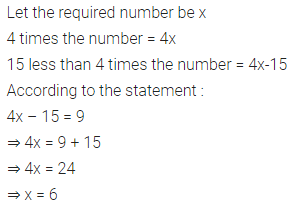Question 2.
If Megha’s age is increased by three times her age, the result is 60 years. Find her age
Solution: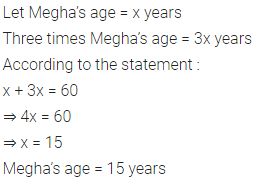Question 3.
28 is 12 less than 4 times a number. Find the number.
Solution:Question 4.
Five less than 3 times a number is -20. Find the number.
Solution:Question 5.
Fifteen more than 3 times Neetu’s age is the same as 4 times her age. How old is she?
Solution: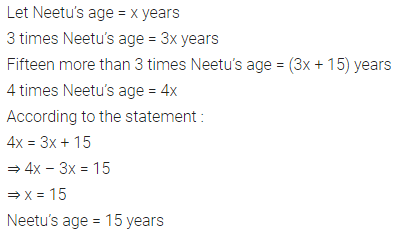Question 6.
A number decreased by 30 is the same as 14 decreased by 3 times the number; Find the number.
Solution:Question 7.
A’s salary is same as 4 times B’s salary. If together they earn Rs.3,750 a month, find the salary of each.
Solution: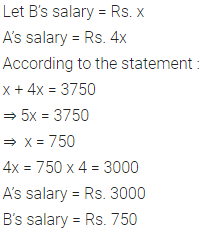Question 8.
Separate 178 into two parts so that the first part is 8 less than twice the second part.
Solution:Question 9.
Six more than one-fourth of a number is two-fifth of the number. Find the number.
Solution:Question 10.
The length of a rectangle is twice its width. If its perimeter is 54 cm; find its length.
Solution: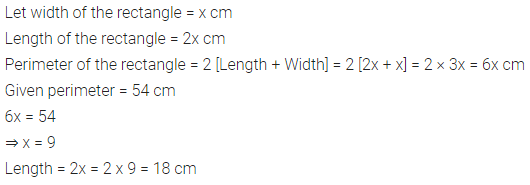Question 11.
A rectangle’s length is 5 cm less than twice its width. If the length is decreased by 5 cm and width is increased by 2 cm; the perimeter of the resulting rectangle will be 74 cm. Find the length and the width of the origi¬nal rectangle.
Solution:Question 12.
The sum of three consecutive odd numbers is 57. Find the numbers.
Solution: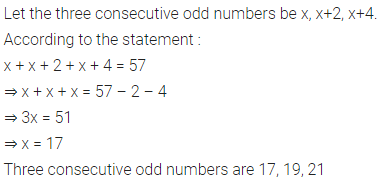Question 13.
A man’s age is three times that of his son, and in twelve years he will be twice as old as his son would be. What are their present ages.
Solution: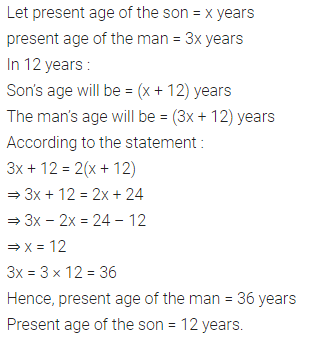Question 14.
A man is 42 years old and his son is 12 years old. In how many years will the age of the son be half the age of the man at that time?
Solution: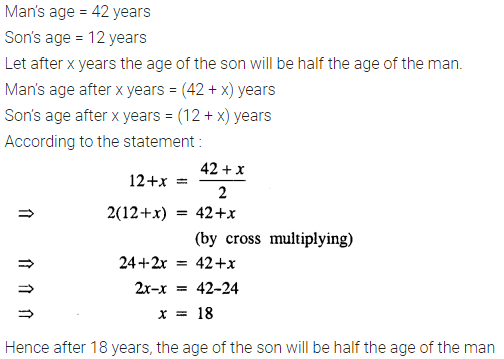Question 15.
A man completed a trip of 136 km in 8 hours. Some part of the trip was covered at 15 km/hr and the remaining at 18 km/hr. Find the part of the trip covered at 18 km/hr.
Solution: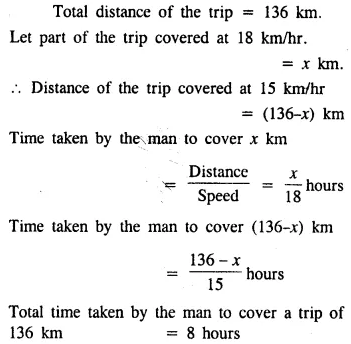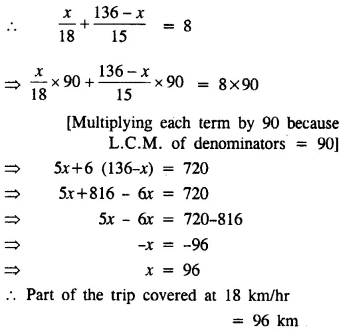Question 16.
The difference of two numbers is 3 and the difference of their squares is 69. Find the numbers.
Solution: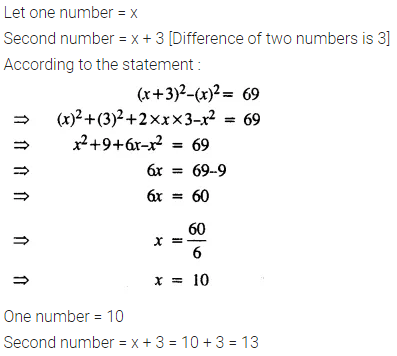Question 17.
Two consecutive natural numbers are such that one-fourth of the smaller exceeds one-fifth of the greater by 1. Find the numbers.
Solution: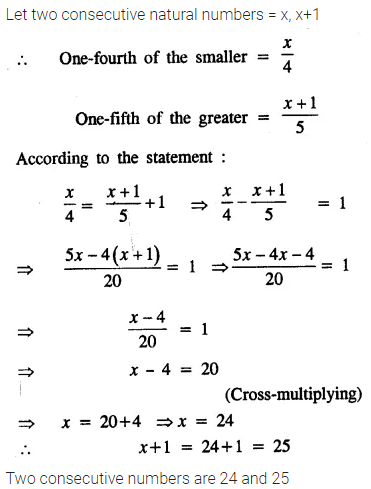Question 18.
Three consecutive whole numbers are such that if they are divided by 5, 3 and 4 respectively; the sum of the quotients is 40. Find the numbers.
Solution: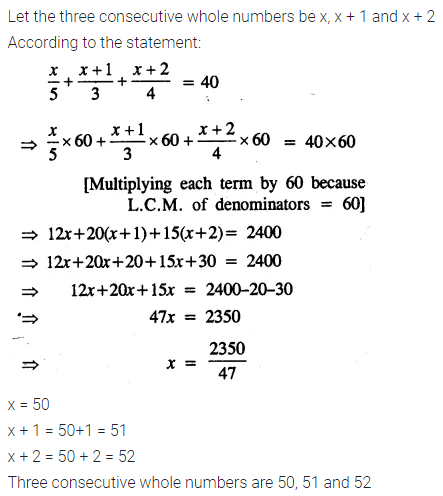Question 19.
If the same number be added to the numbers 5, 11, 15 and 31, the resulting numbers are in proportion. Find the number.
Solution:Question 20.
The present age of a man is twice that of his son. Eight years hence, their ages will be in the ratio 7 : 4. Find their present ages.
Solution:### Linear Equations in one Variable Exercise 14C – Selina Concise Mathematics Class 8 ICSE Solutions

Question 1.
Solve:
(i) $$\frac { 1 }{ 3 } x-6=\frac { 5 }{ 2 }$$
(ii) $$\frac { 2x }{ 3 } -\frac { 3x }{ 8 } =\frac { 7 }{ 12 }$$
(iii) (x + 2)(x + 3) + (x – 3)(x – 2) – 2x(x + 1) = 0
(iv) $$\frac { 1 }{ 10 } -\frac { 7 }{ x } =35$$
(v) 13(x – 4) – 3(x – 9) – 5(x + 4) = 0
(vi) x + 7 – $$\frac { 8x }{ 3 } =\frac { 17x }{ 6 } -\frac { 5x }{ 8 }$$
(vii) $$\frac { 3x-2 }{ 4 } -\frac { 2x+3 }{ 3 } =\frac { 2 }{ 3 } -x$$
(viii) $$\frac { x+2 }{ 6 } -\left( \frac { 11-x }{ 3 } -\frac { 1 }{ 4 } \right) =\frac { 3x-4 }{ 12 }$$
(ix) $$\frac { 2 }{ 5x } -\frac { 5 }{ 3x } =\frac { 1 }{ 15 }$$
(x) $$\frac { x+2 }{ 3 } -\frac { x+1 }{ 5 } =\frac { x-3 }{ 4 } -1$$
(xi) $$\frac { 3x-2 }{ 3 } +\frac { 2x+3 }{ 2 } =x+\frac { 7 }{ 6 }$$
(xii) $$x-\frac { x-1 }{ 2 } =1-\frac { x-2 }{ 3 }$$
(xiii) $$\frac { 9x+7 }{ 2 } -\left( x-\frac { x-2 }{ 7 } \right) =36$$
(xiv) $$\frac { 6x+1 }{ 2 } +1=\frac { 7x-3 }{ 3 }$$
Solution: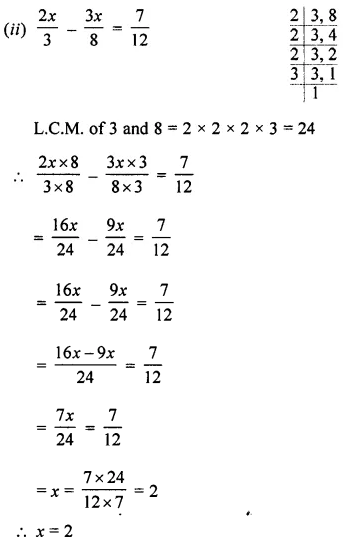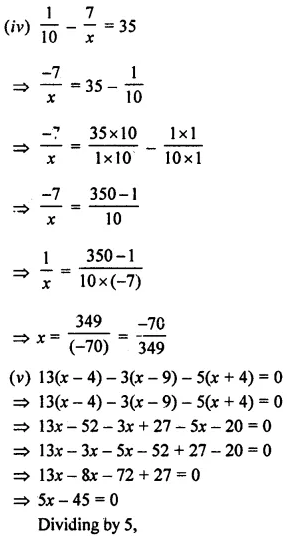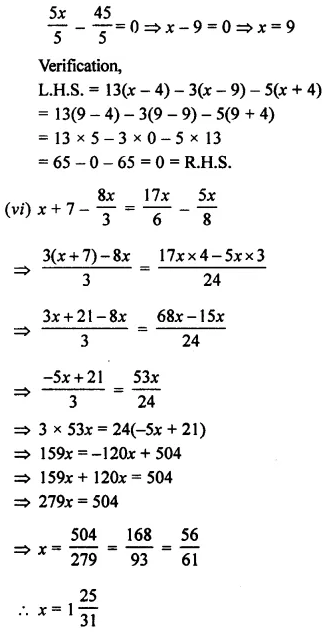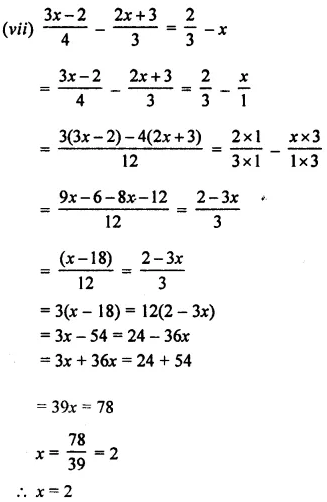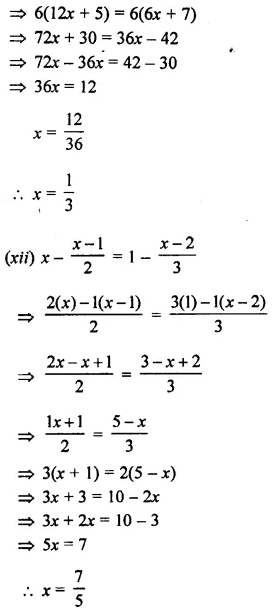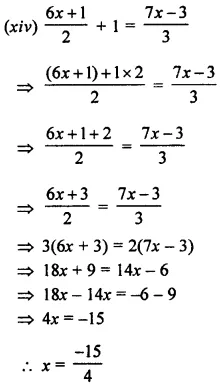Question 2.
After 12 years, I shall be 3 times as old as 1 was 4 years ago. Find my present age.
Solution:Question 3.
A man sold an article for 7396 and gained 10% on it. Find the cost price of the article
Solution: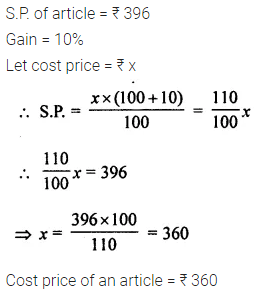Question 4.
The sum of two numbers is 4500. If 10% of one number is 12.5% of the other, find the numbers.
Solution: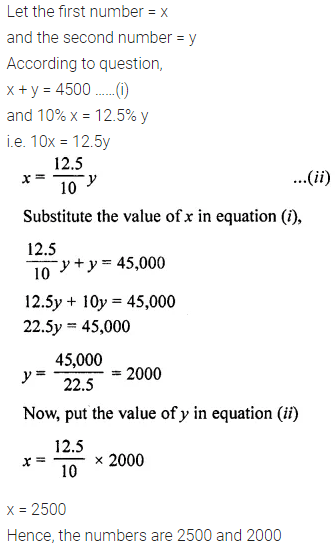Question 5.
The sum of two numbers is 405 and their ratio is 8 : 7. Find the numbers.
Solution: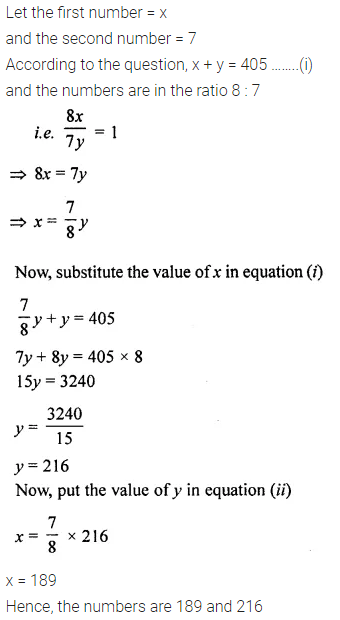Question 6.
The ages of A and B are in the ratio 7 : 5. Ten years hence, the ratio of their ages will be 9 : 7. Find their present ages.
Solution: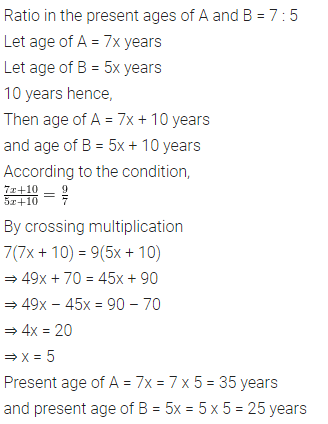Question 7.
Find the number whose double is 45 greater than its half.
Solution: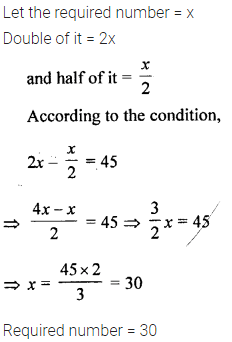Question 8.
The difference between the squares of two consecutive numbers is 31. Find the numbers.
Solution: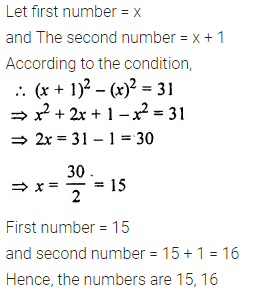Question 9.
Find a number such that when 5 is subtracted from 5 times the number, the result is 4 more than twice the number.
Solution:Question 10.
The numerator of a fraction is 5 less than its denominator. If 3 is added to the numerator, and denominator both, the fraction becomes $$\frac { 2 }{ 3 }$$. Find the original fraction.
Solution: